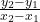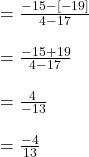## Find the slope of the line through each pair of points using the formula. (17, -19) (4, -15)

Question

Find the slope of the line through each pair of points using the formula.
(17, -19) (4, -15)

in progress 0
3 weeks 2021-08-30T21:02:27+00:00 2 Answers 0 views 0

– 4 / 13

Step-by-step explanation:

Algebra work is easy 🙂

good luckkk

Slope =